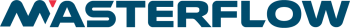## BACK TO LEARNING CENTREPositive Suction Head could be defined as pressure which causes liquid to enter the suction side of a pump. The most common problems encountered in a pumping system are located on the suction side. Each individual pump has a hydraulic requirement at the suction inlet called Nett Positive Suction Head Required (N.P.S.H.R.)

Nett Positive Suction Head is the amount of Suction Head left after various losses have been deducted from a starting point.

The starting point is atmospheric pressure, which is measured in “absolute” terms. Absolute means starting from a perfect vacuum: i.e. at sea level, atmospheric pressure will be about 1 bar abs; 14.5 p.s.i. abs; 100kPa abs, or equivalent to 10.3 metres head of water. (Normal readings of pressure start by taking atmospheric pressure as the zero point and are referred to as “gauge” pressures.)

For every pump system there is a value for the pressure available at the eye of the impeller usually expressed in metres known as: – Nett Positive Suction Head Available (N.P.S.H.A.)

So – to calculate Nett Positive Suction Head Available (N.P.S.H.A.), for any system we use one of two equations depending upon whether the system has a suction lift or flooded suction.

N.P.S.H.A. = Ha + Hs – Hv – Hf, or(flooded suction)

N.P.S.H.A. = Ha – Hl – Hv – Hf, where

Ha = absolute pressure head on liquid surface (frequently atmospheric pressure);

Hs = liquid level above pump centre line.

Hl = liquid level below pump centre line.

Hv = liquid vapour pressure (absolute) at pumping temperature.

Hf = friction losses in pipework.

EXAMPLE: Water at 25 degrees Celsius, suction lift of 2.2 metres, friction

losses totalling 2.15 metres, gives us:

N.P.S.H.A. = 10.37 (Ha) – 2.2 (Hl) – 0.33 (Hv) – 2.15 (Hf)

so N.P.S.H.A. = 5.69 metres.

NOTES: 1. Vapour pressure (Hv) is found from standard tables or given by client.

1. Keep all figures in the same units.

The other consideration is N.P.S.H.R. – Nett Positive Suction Head Required. This is a function of pump design and is usually shown graphically on centrifugal pump curves. Positive displacement pumps also have specific N.P.S.H.R., and this can be shown graphically or by table. N.P.S.H.R. is the lowest N.P.S.H. at which a pump will operate satisfactorily without cavitation (a separate discussion).

In every case N.P.S.H.A. MUST BE GREATER than N.P.S.H.R.

It should be noted that atmospheric pressure varies with altitude, and this needs to be taken into account, since atmospheric pressure is often our starting point (Ha).

Examples of this variation are listed below.

 Altitude Atmospheric Pressure (kPa Absolute) Equivalent Head (Metres of Water) Feet Metres 0 0 101.3 10.37 500 152.4 99.2 10.19 1000 304.8 97.8 10.00 1500 457.2 95.7 9.82 2000 609.6 94.4 9.64 2500 762.0 92.3 9.45

## BACK TO ALL NEWS